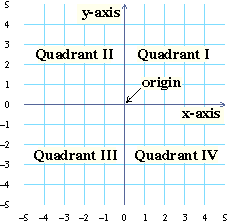# Points and Coordinates

The coordinates of a point give us directions so that we can locate it on a coordinate plane. In this lesson you will learn to describe points in the plane and study the different parts of the coordinate plane.A point has coordinates \$(x, y)\$, where \$x\$ is given by the labels below the coordinate grid, and \$y\$ is given by the labels to the left of the coordinate grid.

There is a red point on the top coordinate grid to the left. What are the point’s coordinates? The point you type in will be illustrated on the bottom grid.
\$x\$\$y\$
Fill in the missing \$y\$ values in this table so that the points on the bottom grid to the left look like the ones on the top grid.
\$x\$\$y\$
Complete this table so that the points on the bottom grid to the left look like the points on the top grid.
\$x\$\$y\$
Complete this table so that the points on the bottom grid to the left look like the points on the top grid.
\$x\$\$y\$
\$2\$\$2\$
\$3\$\$2\$
\$3\$
\$3\$
\$4\$

Sometimes we want to name several variables in a way that shows they are related. When we do, we’ll write a variable with a subscripted number. For example, \$x_1\$ (pronounced “\$x\$-1” or “\$x\$-sub-1”) is a variable which is related to (but different from) the variable \$x\$.

Use the slider to the left to change the value of \$x_1\$ for the point \$(x_1,2)\$. Notice how the point moves.

 Does the point move horizontally (side to side) or vertically (up and down) as you change \$x_1\$?
 Does the point move to the right or to the left as \$x_1\$ increases?
Slide \$x_1\$ to each value given here and complete the table.
\$x_1\$\$x\$-coordinate \$y\$-coordinate
\$2\$\$2\$\$2\$
\$4\$
\$-3\$
 What is the relationship between \$x_1\$ and the \$x\$-coordinate of the point? Are they the same or are they different?

Use the slider to change the value of \$y_1\$ and notice how the point \$(2,y_1)\$ moves.

 Does the point move horizontally or vertically as you change \$y_1\$?
 Does the point move up or down as \$y_1\$ increases?
Use the slider to complete this table.
\$y_1\$\$x\$-coordinate\$y\$-coordinate
\$2\$
\$4\$
\$-3\$
 What is the relationship between \$y_1\$ and the \$y\$-coordinate of the point? Are they the same or are they different?

Use the \$x_1\$ slider to change the \$x\$-coordinate of the point \$(x_1,y_1)\$. Use the \$y_1\$ slider to change the \$y\$-coordinate of the point. Notice how the point moves. The coordinates of the point are shown below the grid.

 Use the sliders to find the coordinates of the origin. The diagram from the top of this page is repeated below; it shows the location of the origin, as well as the other features of the coordinate grid you will be asked about in this question.Use the sliders to find the point \$(2,0)\$. Notice that this point is on the \$x\$-axis. Give the coordinates of two more points on the \$x\$-axis.
 Use the sliders to find the point \$(0,2)\$. Notice that this point is on the \$y\$-axis. Give the coordinates of two more points on the \$y\$-axis.
 What axis do you think the point \$(5,0)\$ is on? Use the sliders to check your answer.